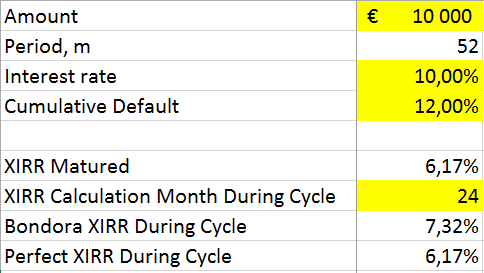# How does Bondora calculate the net return?

We use the XIRR function (extended internal rate of return) to calculate the net return. XIRR is used to calculate the internal rate of return for a schedule of cash flows (repayments minus initial investment) that are neither necessarily periodic (payments are not made on a single day each month) nor always positive (portfolio has both outgoing and incoming payments).

Internal rate of return is a discount rate that makes the net present value (NPV) of all cash flows from a particular portfolio equal to zero. Discounting cash flow means that you are reducing the future cash flow projections to arrive at its present value (how much 100 Euro a year from now is worth today). This is mathematically done by dividing the future cash flow by 1 plus internal rate of return in the power of the fractional period (e.g. if you are using an annual internal rate of return and a payment is 4 months from now then you would take 1 plus internal rate of return to the power of 4/12).

XIRR uses the loan issue date and amount, loan actual repayment dates and amounts and sum of scheduled future principal repayments (this is assumed by us to equal the present value of the portfolio) to calculate the internal rate of return of your investment portfolio on Bondora. This approach writes off all overdue and unpaid principal and interest payments immediately from the calculation. No assumptions for future interest payments or loss of provisions are made as this is expected to be covered within our approach to arrive at the present value of the portfolio.

In other words, if you would borrow money at a rate equal to the net return shown on Bondora in order to finance your investments you would end up breaking even. If you are borrowing money at a lower rate or your alternative investment opportunities (e.g. mutual fund) have a lower rate of return, then you are making a profit compared to other options and it makes sense to continue or increase investment.Bondora XIRR calculation model